## 设计时间序列 NARX 反馈神经网络

NARX 模型的定义方程是

`$y\left(t\right)=f\left(y\left(t-1\right),y\left(t-2\right),\dots ,y\left(t-{n}_{y}\right),u\left(t-1\right),u\left(t-2\right),\dots ,u\left(t-{n}_{u}\right)\right)$`NARX 网络有许多应用。它可以用作预测器，预测输入信号的下一个值。它也可用于非线性滤波，其中目标输出是输入信号的无噪声版本。NARX 网络可用于另一个重要应用，即非线性动态系统的建模。Use the NARMA-L2 Controller Block中开始描述的磁悬浮系统是一个 NARX 网络示例。下图中的底部图显示施加到电磁铁上的电压，顶部图显示永磁体的位置。以 0.01 秒的采样间隔采集数据，形成两个时间序列。```load magdata y = con2seq(y); u = con2seq(u); ```

```d1 = [1:2]; d2 = [1:2]; narx_net = narxnet(d1,d2,10); narx_net.divideFcn = ''; narx_net.trainParam.min_grad = 1e-10; [p,Pi,Ai,t] = preparets(narx_net,u,{},y); ```

（请注意，`y` 序列被视为反馈信号，它既是输入也是输出（目标）。稍后，当您实现闭环时，适当的输出将连接到适当的输入。）现在您已准备好训练网络。

```narx_net = train(narx_net,p,t,Pi); ```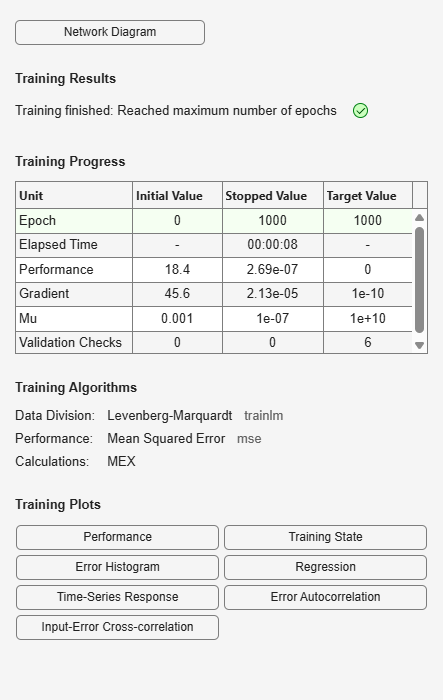```yp = sim(narx_net,p,Pi); e = cell2mat(yp)-cell2mat(t); plot(e) ```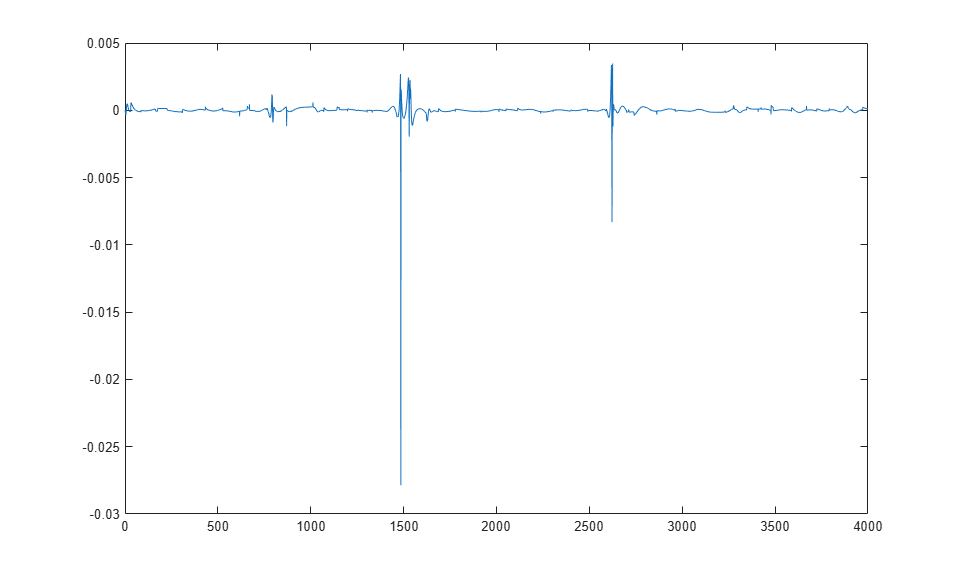```narx_net_closed = closeloop(narx_net); ```

```view(narx_net) ```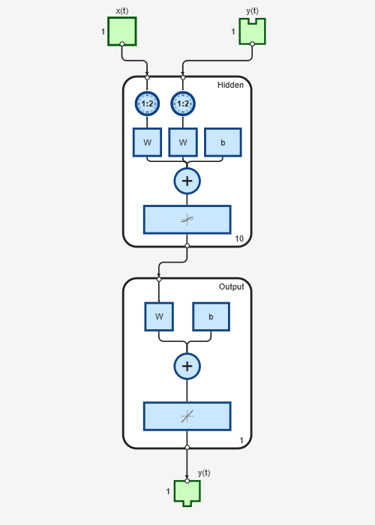```view(narx_net_closed) ```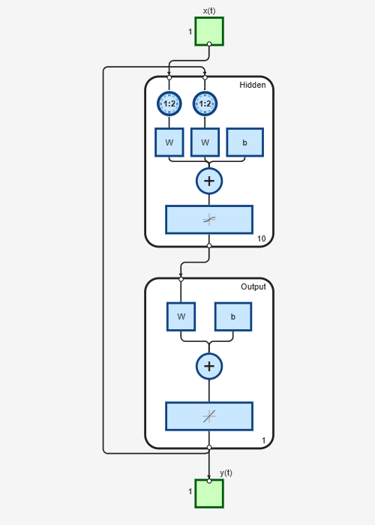```y1 = y(1700:2600); u1 = u(1700:2600); [p1,Pi1,Ai1,t1] = preparets(narx_net_closed,u1,{},y1); yp1 = narx_net_closed(p1,Pi1,Ai1); TS = size(t1,2); plot(1:TS,cell2mat(t1),'b',1:TS,cell2mat(yp1),'r') ```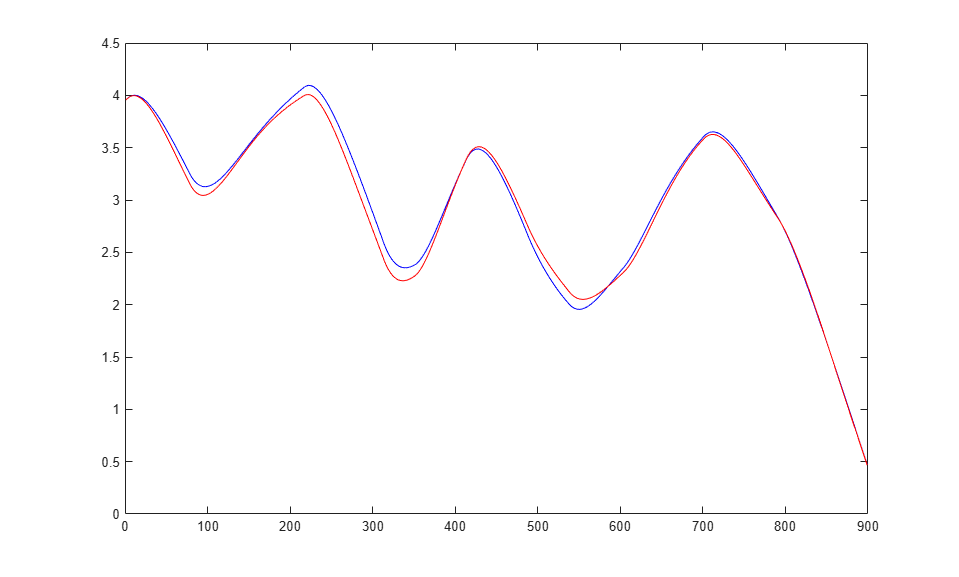### 多个外部变量

`[X,T] = ph_dataset;`

```net = narxnet(10); [x,xi,ai,t] = preparets(net,X,{},T); net = train(net,x,t,xi,ai); y = net(x,xi,ai); e = gsubtract(t,y); ```